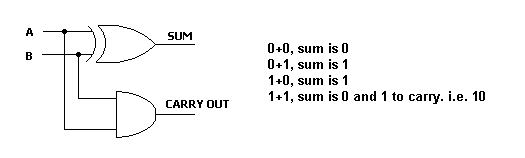Home > Electronics Tutorials > Combination Logic > The Half Adder Tutorial

# Combination Logic TutorialThis adds two 1 bit numbers.

The table shows the outputs.

When A and B are both 0, the sum is 0.

When one is 0 and the other is 1 then the sum is 1.

When both are 1 then the sum is 0 and carry output is 1.